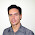## Thursday, December 27, 2012

### Measure light (lux) with LDR on AVR Atmega using LDR library 01

A photoresistor or light dependent resistor (LDR) is a resistor whose resistance decreases with increasing incident light intensity; in other words, it exhibits photoconductivity.
The lux (symbol: lx) is the SI unit of illuminance and luminous emittance, measuring luminous flux per unit area.

This library implements a way to estimate lux given a LDR resistance value read from an ADC input pin of an AVR Atmega.
The estimation is based on this formula:
lux = A*((R/B)^C)
where R is the LDR resistance.
An Excel spreadsheet is provided to help the estimation of A, B and C values. You have to read lux values using a calibrated luxmeter, and the R values of your LDR, write it down on the Excel sheet, and estimate A, B and C, power regression can help you estimating values.

Code

Notes

1.lux = A*((R/B)^C)
What's meaning in above formula

1.Hello, that's a power regression.R is the LDR resistance, A, B and C are values dependant on the LDR model.

2.Hello Davide,

I'm Song and nice to meet you. May I ask you for a few question? please.

Could you suggest me how to estimate the A, B, C and formula?

Thank you so much,
Song.

1.Hello.
Write down some values read from a calibrated lux meter. And the values of the resistence of your LDR exposed to the same amount of light of the lux meter sensor. Then, you could use matlab and do power regression, or simply put the value you read in the spreasdheet provided and by hand try to find the best A, B, C parameter to fit the curve for your LDR. Hope this helps.

2.Hello Davide,

3.